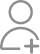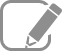Learn MATLAB from the Best Tutors

•Affordable fees
•1-1 or Group class
•Flexible Timings
•Verified Tutors

Search in

# When I enter the following code x= [3,11,7,0,-1]; stem(x) in the MATLAB . Why the figure doesn't show the x(1) =3? What's wrong?Follow 1AnswerDefine axis in your code. x axis starting from 0 to 5 or 6 and y axis -1 to 11 or 12. Then, You will be able to see x(1)=3. axis()

Related Questions

How to implement multi-user mimo with OFDM
MIMO itself is a multi user system. in MIMO OFDM Multiple transmitters and receivers have to be implemented in order to build an efficient communication system, which uses multiple antennas. Its easy to...I am a student living in Turkey, am doing master degree in computer engineering, currently am writing my thesis, doing Image Fusion, I was working the implementation of a couple of algorithms, most of them I finished, must am facing some difficulties on some algorithms, so I wonder if you have some one who is experienced in image fusion algorithm, if that person is available I am ready to pay him, so please let me know if it's posible
I am interested in this. I have successfully completed a project in Image Fusion for company called as Project Deals, Based on IEEE Paper: This project involves fusing the general or medical images from...I am doing my M.tech in electronics and communications and I have some problem in generating my MIMO OFDM system model in MATLAB. Anyone interested kindly help.
Will definitely help if needed.Would you recommend any good books to get an introduction to MATLAB?
Matlab is one of the most powerful simulation software. There are many textbooks that teach you how to use it depending on your research interest but they start all by talking about the main functions...I am interested to learn simulink product in matlab. Anyone help me please.
Kindly go through my profileNow ask question in any of the 1000+ Categories, and get Answers from Tutors and Trainers on UrbanPro.com

Related Lessons

A trigonometric identity is given by: cos^2(x/2)=(tanx+sinx)/2tanx
A trigonometric identity is given by: cos^2(x/2)=(tanx+sinx)/2tanx We would calculate each side of the equation, substituting.x=pi/2 sol. >> x=pi/5; LHS=cos(x/2)^2 LHS = 0.9045 RHS=(tan(x)+sin(x))/(2*tan(x)) RHS = 0.9045Find MATLAB Training near you

Looking for MATLAB Training?

Learn from the Best Tutors on UrbanPro

Are you a Tutor or Training Institute?

Join UrbanPro Today to find students near you
X

### Looking for MATLAB Classes?

The best tutors for MATLAB Classes are on UrbanPro

• Select the best Tutor
• Book & Attend a Free Demo
• Pay and start Learning### Learn MATLAB with the Best Tutors

The best Tutors for MATLAB Classes are on UrbanPro